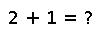# mod2pi

mod2pi(x)

Modulus after division by 2pi, returning in the range [0,2pi).

This function computes a floating point representation of the modulus after division by numerically exact 2pi, and is therefore not exactly the same as mod(x,2pi), which would compute the modulus of `x` relative to division by the floating-point number 2pi.

## Examples

``````julia> mod2pi(8)
1.7168146928204135

julia> mod2pi(2pi)
6.283185307179586``````

Checking you are not a robot: